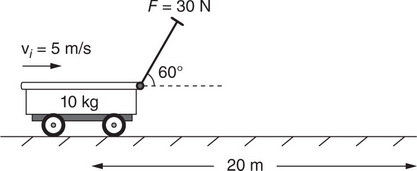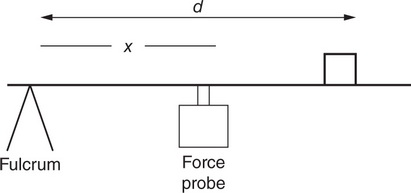# AP Physics 1 Practice Test 13

### Test Information10 questions18 minutes

1.Three wagons each have the same total mass (including that of the wheels) and four wheels, but the wheels are differently styled. The structure, mass, and radius of each wagon’s wheels are shown in the preceding chart. In order to accelerate each wagon from rest to a speed of 10 m/s, which wagon requires the greatest energy input?

2. A swimmer is able to propel himself forward through the water by moving his arms. Which of the following correctly states the applicant and recipient of the force responsible for the swimmer’s forward acceleration?

3.A 10-kg wagon moves horizontally at an initial speed of 5 m/s. A 30-N force is applied to the wagon by pulling the rigid handle, which is angled 60° above the horizontal. The wagon continues to move horizontally for another 20 m. A negligible amount of work is converted into thermal energy. By how much has the wagon’s kinetic energy increased over the 20 m?

4. A moving 1.5-kg cart collides with and sticks to a 0.5-kg cart which was initially at rest. Immediately after the collision, the carts each have the same ______ as each other.

5.Four identical resistors are connected to a battery in the configuration shown in the preceding figure.

Which of the following ranks the current I through each resistor?

6.Four identical resistors are connected to a battery in the configuration shown in the preceding figure.

Which graph represents the electric potential with respect to the negative end of the battery as a function of the location on a loop of wire starting from the positive end of the battery, going through resistors 1, 2, and 4, and ending back on the negative end of the battery?

7.A force probe is used to stretch a spring by 20 cm. The graph of the force as a function of distance shown in the preceding figure is produced and used to determine the amount of work done in stretching the spring 20 cm. The experimenter reports the result as 3,000 N·cm. Which of the following is a reasonable estimate of the experimental uncertainty on this measurement?

8. A string of fixed tension and linear mass density is attached to a vibrating speaker. It is observed that a speaker frequency of 60 Hz does not produce standing waves in the string. Which explanation for this phenomenon is correct?

9.In the laboratory, a long platform of negligible mass is free to rotate on a fulcrum. A force probe is placed a fixed distance x from the fulcrum, supporting the platform. An object of fixed mass is placed a variable distance d from the fulcrum. For each position d, the force probe is read. It is desired to determine the mass of the object from a graph of data. Which of the following can determine the object’s mass?

10. In Collision A, two carts collide and bounce off each other. In Collision B, a ball sticks to a rigid rod, which begins to rotate about the combined center of mass. Which of the following statements about quantities in each collision is correct?